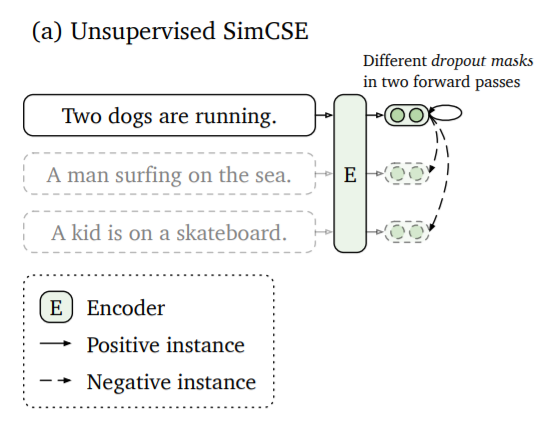# SimCSE¶

Gao et al. present in SimCSE a simple method to train sentence embeddings without having training data.

The idea is to encode the same sentence twice. Due to the used dropout in transformer models, both sentence embeddings will be at slightly different positions. The distance between these two embeddings will be minized, while the distance to other embeddings of the other sentences in the same batch will be maximized (they serve as negative examples).## Usage with SentenceTransformers¶

SentenceTransformers implements the MultipleNegativesRankingLoss, which makes training with SimCSE trivial:

```from sentence_transformers import SentenceTransformer, InputExample
from sentence_transformers import models, losses
from torch.utils.data import DataLoader

# Define your sentence transformer model using CLS pooling
model_name = 'distilroberta-base'
word_embedding_model = models.Transformer(model_name, max_seq_length=32)
pooling_model = models.Pooling(word_embedding_model.get_word_embedding_dimension())
model = SentenceTransformer(modules=[word_embedding_model, pooling_model])

# Define a list with sentences (1k - 100k sentences)
train_sentences = ["Your set of sentences",
"Model will automatically add the noise",
"And re-construct it",
"You should provide at least 1k sentences"]

# Convert train sentences to sentence pairs
train_data = [InputExample(texts=[s, s]) for s in train_sentences]

# DataLoader to batch your data
train_dataloader = DataLoader(train_data, batch_size=128, shuffle=True)

# Use the denoising auto-encoder loss
train_loss = losses.MultipleNegativesRankingLoss(model)

# Call the fit method
model.fit(
train_objectives=[(train_dataloader, train_loss)],
epochs=1,
show_progress_bar=True
)

model.save('output/simcse-model')
```

## SimCSE from Sentences File¶

train_simcse_from_file.py loads sentences from a provided text file. It is expected, that the there is one sentence per line in that text file.

SimCSE will be training using these sentences. Checkpoints are stored every 500 steps to the output folder.

## Ablation Study¶

We use the evaluation setup proposed in our TSDAE paper.

Using mean pooling, with max_seq_length=32 and batch_size=128

Base Model AskUbuntu Test-Performance (MAP)
distilbert-base-uncased 53.59
bert-base-uncased 54.89
distilroberta-base 56.16
roberta-base 55.89

Using mean pooling, with max_seq_length=32 and distilroberta-base model.

Batch Size AskUbuntu Test-Performance (MAP)
128 56.16
256 56.63
512 56.69

Using max_seq_length=32, distilroberta-base model, and 512 batch size.

Pooling Mode AskUbuntu Test-Performance (MAP)
Mean pooling 56.69
CLS pooling 56.56
Max pooling 52.91

Note: This is a re-implementation of SimCSE within sentence-transformers. For the official CT code, see: princeton-nlp/SimCSE